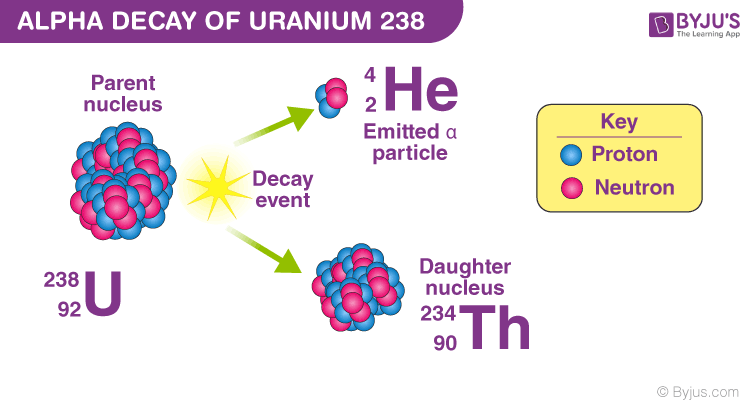# Alpha Decay

Alpha decay is a nuclear decay process where an unstable nucleus changes to another element by shooting out a particle composed of two protons and two neutrons. This ejected particle is known as an alpha particle. Ernest Rutherford distinguished alpha decay from other forms of radiation by studying the deflection of the radiation through a magnetic field. The deflection of alpha decay would be a positive charge as the particles have a +2e charge. In this article, you will study alpha decay in detail.

## What is Alpha Decay?

Alpha decay or α-decay is a type of radioactive decay in which the atomic nucleus emits an alpha particle thereby transforming or decaying into a new atomic nucleus. Here the atomic mass number of the newly formed atom will be reduced by four and the atomic number will be reduced by two. The emitted alpha particle is also known as a helium nucleus. The mass of the alpha particles is relatively large and has a positive charge.

Ernest Rutherford distinguished alpha decay from other forms of radiation by studying the deflection of the radiation through a magnetic field. The deflection of alpha decay would be a positive charge as the particles have a +2e charge.

### Alpha Decay Equation

In α-decay, the mass number of the product nucleus (daughter nucleus) is four less than that of the decaying nucleus (parent nucleus), while the atomic number decreases by two. In general, the alpha decay equation is represented as follows:

$$\begin{array}{l}_{Z}^{A}\textrm{X}\rightarrow _{Z-2}^{A-4}\textrm{Y}+_{2}^{4}\textrm{He}\end{array}$$

where,

• $$\begin{array}{l}_{Z}^{A}\textrm{X} \textup{ is the parent nucleus}\end{array}$$
• A is the total no.of nucleons
• Z is the total no.of protons
• $$\begin{array}{l}_{Z-2}^{A-4}\textrm{Y} \textup{ is the daughter nucleus}\end{array}$$
• $$\begin{array}{l}_{2}^{4}\textrm{He} \textup{ is the released alpha particle}\end{array}$$

### Alpha Decay Example

A well-known example of alpha decay is the decay of uranium.

$$\begin{array}{l}_{92}^{238}\textrm{U} \textup{ to thorium } _{90}^{234}\textrm{Th} \textup{ with the emission of a helium nucleus } _{2}^{4}\textrm{He}.\end{array}$$
$$\begin{array}{l}_{92}^{238}\textrm{Ur}\rightarrow _{90}^{234}\textrm{Th}+_{2}^{4}\textrm{He}\end{array}$$Other examples of alpha decay include:

$$\begin{array}{l}_{93}^{237}\textrm{Np}\rightarrow _{91}^{233}\textrm{Pa}+_{2}^{4}\textrm{He}\end{array}$$
$$\begin{array}{l}_{78}^{175}\textrm{Pt}\rightarrow _{76}^{171}\textrm{Os}+_{2}^{4}\textrm{He}\end{array}$$
$$\begin{array}{l}_{64}^{149}\textrm{Gd}\rightarrow _{62}^{145}\textrm{Sm}+_{2}^{4}\textrm{He}\end{array}$$

## What happens in Alpha Decay?

In alpha decay, the nucleus emits an alpha particle or a helium nucleus. Alpha decay occurs in massive nuclei that have a large proton to neutron ratio. Alpha radiation minimizes the protons to neutrons ratio in the parent nucleus, thereby bringing it to a more stable configuration.

As an example, let us consider the decay of 210Po by the emission of an alpha particle.

Polonium nucleus has 84 protons and 126 neutrons, therefore the proton to neutron ratio is Z/N = 84/126, or 0.667. On the other hand, 210Pb nucleus has 82 protons and 124 neutrons, thereby resulting in a ratio of 82/124, or 0.661. This small change in the Z/N ratio is enough to put the nucleus into a more stable state (into the region of stable nuclei in the Chart of the Nuclides.)

## Gamow Theory of Alpha Decay

The Geiger–Nuttall law or Geiger–Nuttall rule relates to the decay constant of a radioactive isotope with the energy of the alpha particles emitted. This relation also states that half-lives are exponentially dependent on decay energy, so that very large changes in half-life make comparatively small differences in decay energy, and thus alpha particle energy.

As per this rule, short-lived isotopes emit more energetic alpha particles than long-lived ones. This law was stated by Hans Geiger and John Mitchell Nuttall in the year 1911, hence the name was dedicated to these physicists.

## Frequently Asked Questions – FAQs

Q1

### What is the atomic mass number of the emitted Alpha particle?

The atomic mass number of the emitted Alpha particle is four.
Q2

### How many protons are present in the alpha particle?

Two protons are present in the alpha particle.
Q3

### How many neutrons are present in the alpha particle?

Two neutrons are present in the alpha particle.
Q4

### What is the penetration depth of an Alpha particle?

The penetration power of Alpha rays is low. Generally few centimetres of air or by the skin.
Q5

### What is the polarity of charge of the Alpha Particle?

The emitted Alpha particle is positively charged.
Q6

### What is the average Kinetic Energy of the emitted Alpha Particle?

The average Kinetic energy of the emitted Alpha particle is approximately 5MeV.
Q7

### What is the velocity of the Alpha particle?

The average velocity of the emitted Alpha particle is in the vicinity of 5% of that of c.

The spontaneous decay or breakdown of an atomic nucleus is known as Radioactive Decay. This decay in a nucleus causes the release of energy and matter from the nucleus.

The most common forms of Radioactive decay are:

• Alpha Decay (Helium nucleus is emitted)
• Beta Decay (Electrons are emitted)
• Gamma Decay (High energy photons are emitted)

The articles on these concepts are given below in the table for your reference:

 Related Articles:

### Watch the video and understand the three rules of electronic configuration!Stay tuned to BYJU’S and Fall in Love with Learning!

Test your knowledge on Alpha Decay